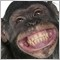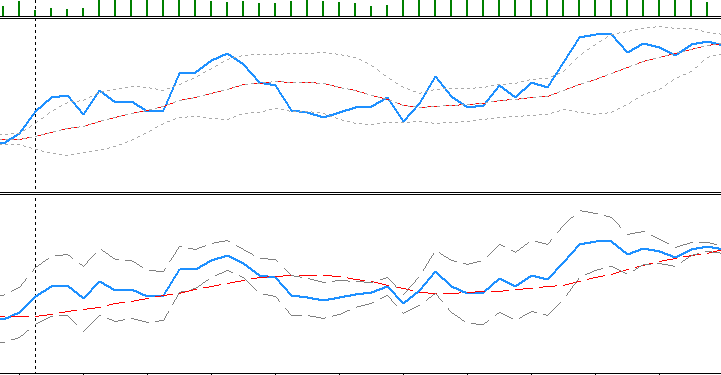Help.. moving indicator from MT4 to MT5 iBands46

Hi there

Can anyone help? I am trying to move my custom indicator from MT4 to MT5 and finding it a very steep learning curve.  I have the first part of the indicator working great but am struggling to get Bollinger Bands running on the signal that it generates! I can drag the bands indicator on manually, looks great, however I cannot create signals from this. I have tried finding an include, writing the formula myself and now trying to call iBands() from within the indicator itself, loading the results into an array. Here is what I have:

BandsHandle=iBands(NULL,0,0,MAvariable,StandardDevVariable,SignalHandle);
CopyBuffer(BandsHandle,1,0,0,ind3);

SignalHandle is the handle of my main buffer (ind1) but I am not getting the handle correctly. Is it possible to get the handle for your first indicator buffer?

I have gone through several articles on how to do this and dissected several other indicators but cannot seem to get bollinger bands or StDev on my indicator. What am I missing.413

Hi!

Your 4th parameter of CopyBuffer is zéro, so you are copying nothing.

Here is an example for getting the current bar:

if(CopyBuffer(BolBandsHandle,0,0,1,BBMidle)<0 || CopyBuffer(BolBandsHandle,1,0,1,BBUp)<0
|| CopyBuffer(BolBandsHandle,2,0,1,BBLow)<0)
{
Alert("Error copying Bollinger Bands indicator Buffers - error:",GetLastError(),"!!");
return;
}46

twalk:

Hi!

Your 4th parameter of CopyBuffer is zéro, so you are copying nothing.

Here is an example for getting the current bar:

Thanks Twalk, I was going with Zero as I was hoping to copy the whole array in one go. I think I am doing something fundamentally wrong. I am trying to find the standard deviation of the output of my indicator. Using iBands was a way to achieve this as it offers exactly that. In attempting to use it I have come across two problems. Can I copy the whole array at once AND how do I get the handle for my calculated buffer? (In this instance the handle I am trying to get is for ind1).

As an alternative I have found the stdev function used by some of the other indicators and tried referencing it myself. Commented in the bottom loop. It doesnt seem to give me the correct values and an out-of-bounds error when I try to reference it in an EA.

Apologies if all this seems very basic, C is a pretty steep learning curve and I have spent several evenings on this now, its driving me slightly crazy!!!

//+------------------------------------------------------------------+
//| Custom indicator initialization function                         |
//+------------------------------------------------------------------+
int OnInit()
{
//--- indicator buffers mapping
SetIndexBuffer(0,ind1,INDICATOR_DATA);
SetIndexBuffer(1,ind2,INDICATOR_DATA);
SetIndexBuffer(2,ind3,INDICATOR_DATA);
SetIndexBuffer(3,ind4,INDICATOR_DATA);
//SetIndexBuffer(5,distance,INDICATOR_CALCULATIONS);
//---
return(0);
}
//+------------------------------------------------------------------+
//| Custom indicator iteration function                              |
//+------------------------------------------------------------------+
int OnCalculate(const int rates_total,
const int prev_calculated,
const datetime &time[],
const double &open[],
const double &high[],
const double &low[],
const double &close[],
const long &tick_volume[],
const long &volume[],
{
//---
ArraySetAsSeries(time,true);ArraySetAsSeries(open,true);ArraySetAsSeries(high,true);ArraySetAsSeries(low,true);

ArraySetAsSeries(ind1,true);
ArraySetAsSeries(ind2,true);
ArraySetAsSeries(ind3,true);
ArraySetAsSeries(ind4,true);

MqlRates rates1[]; ArraySetAsSeries(rates1,true);
MqlRates rates2[]; ArraySetAsSeries(rates2,true);

if(prev_calculated<rates_total)
{
for(int i=0;i<rates_total;i++)
{

CopyRates(Symbol2,0,time[i],1,rates1);                //rates for GBP/USD
CopyRates(Symbol(),0,time[i],1,rates2);               //rates for GBP/EUR

//--- middle line
ind1[i]=(rates1.close) / (rates2.close);        //price ratio to first indicator
}
SimpleMAOnBuffer(rates_total,prev_calculated,0,MA,ind1,ind2);   //ma of price ratio

BandsHandle=iBands(NULL,0,MA,0,StandardDev,8);
CopyBuffer(BandsHandle,1,0,0,ind3);

for(int i=0;i<rates_total;i++)  //Loop to calculate StDev Upper and Lower on ind1 (Not working)
{
// double CurrMovAverage = SimpleMA(i+MA,MA,ind1);
// ind2[i] = CurrMovAverage;
//  double CurrStDev = StdDev_Func(i,ind1,ind2,MA);
//  ind3[i] = ind1[i]+(CurrStDev);
//  ind4[i] = ind1[i]-(CurrStDev);
}

}

//--- return value of prev_calculated for next call
return(rates_total);
}

double StdDev_Func(int position,const double &price[],const double &MAprice[],int period)
{
//--- variables
double StdDev_dTmp=0.0;
//--- check for position
if(position<period) return(StdDev_dTmp);
//--- calcualte StdDev
for(int i=0;i<period;i++) StdDev_dTmp+=MathPow(price[position-i]-MAprice[position],2);
StdDev_dTmp=MathSqrt(StdDev_dTmp/period);
//--- return calculated value
return(StdDev_dTmp);
}413

You won't be able to pass ind1 to iBands. Only possibility is your StdDev_Func()

But I don't understand the intention in your nested loops. You are re-declaring the value of i at each step of the main loop. Normally you should have a compilation error.

At least it would be:

if(prev_calculated<rates_total)
{
for(int i=0;i<rates_total;i++)
{

CopyRates(Symbol2,0,time[i],1,rates1);                //rates for GBP/USD
CopyRates(Symbol(),0,time[i],1,rates2);               //rates for GBP/EUR

//--- middle line
ind1[i]=(rates1.close) / (rates2.close);        //price ratio to first indicator
}
SimpleMAOnBuffer(rates_total,prev_calculated,0,MA,ind1,ind2);   //ma of price ratio

BandsHandle=iBands(NULL,0,MA,0,StandardDev,8);
CopyBuffer(BandsHandle,1,0,0,ind3);

double CurrMovAverage = SimpleMA(j+MA,MA,ind1);
ind2[j] = CurrMovAverage;
double CurrStDev = StdDev_Func(j,ind1,ind2,MA);
ind3[j] = ind1[j]+(CurrStDev);
ind4[j] = ind1[j]-(CurrStDev);

}
Maybe you don't need this second loop.46

twalk:

You won't be able to pass ind1 to iBands. Only possibility is your StdDev_Func()

But I don't understand the intention in your nested loops. You are re-declaring the value of i at each step of the main loop. Normally you should have a compilation error.

At least it would be:

Maybe you don't need this second loop.

Hi Twalk,

Firstly, thank you very much for your help. After a bit of a break I have come back to this and thanks to your help it feels like I am really getting somewhere.

Having the CurrMovingAverage code in the same loop as the Price ratio one seems to cause a lot of problems. Sometimes the chart loads correctly, other times I get some crazy results. So I have moved it into the second (J) loop and it works great. Adding in the StDev formula's I have had some limited success.

Here is my code

if(prev_calculated<rates_total)
{
for(int i=0;i<rates_total;i++)
{

CopyRates(Symbol2,0,time[i],1,rates1);                //rates for GBP/USD
CopyRates(Symbol(),0,time[i],1,rates2);               //rates for GBP/EUR

//--- middle line
ind1[i]=(rates1.close) / (rates2.close);        //price ratio to first indicator

//---- MA code in here gives random crazy results!

}

for(int j=0;j<rates_total;j++)
{
//------ moving it here works very well
double CurrMovAverage=SimpleMA(j+MA,MA,ind1);
ind2[j]= CurrMovAverage;

//------ These results print great but don't seem correct

double CurrStDev = StdDev_Func(j,ind1,ind2,MA);
ind3[j] = ind1[j]+CurrStDev;
ind4[j] = ind1[j]-CurrStDev;
}

}

But my results are as follows (Top chart is Price Ratio and MA correct with Bollinger Band indicator added manually, bottom is the new code)It seems I am extremely close now. I have tried applying the StDev function to the MA buffer instead and my results seem closer but still not correct. Any ideas.

Again, sorry for if this is all obvious, still trying to understand it here. Really appreciate the help!!

Rich